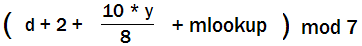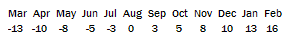## Calculate the day of the week you were born on – fake being an autistic savant!

maths Add comments

My friend and I were curious and we can now do the party trick of figuring out what day of the week a birthday falls on.

For the impatient, here’s the formula :Where d is the day, y is the last 2 digits of your birth year (throw away any remainder of the division) and mlookup is a lookup table :And the result is 0 = Sunday, 1 = Monday, etc

I was watching a show about Daniel Tammet, an autistic savant, and telling my friend Dave about it. One of the things that the media often focus on is how these savants can calculate the day you were born on.

I’ve always suspected that it can’t be that hard to do – there must be some formula for it – and I said so to Dave.

So we got curious and went to find the formula. Sure enough we found one.

At first it looked a bit long-winded. But after a bit of wrangling, we got it down to it’s bare essence with a couple of shortcuts (ie lookup tables in programmer-speak). Now we can do the calculations in our head. It’s still a bit of mental effort but nothing too onerous with a bit of practise.

We got the original formula from here :

http://www.tondering.dk/claus/cal/node3.html#SECTION00360000000000000000

And you can check your answers here :

http://homepages.rootsweb.com/~sdavnprt/bday.htm

Here is an example – 18th December 1977 gives

(18 + 2 + (10 * 76)/8 + 10 ) mod 7

A quick way of dividing by 8 is to divide in half 3 times, so 770/8 = 385/4 = 192/2 = 96.

18 + 2 + 96 + 10 = 126.

126 mod 7 = 0

So 18th December 1977 was a Sunday!

PS I’m in no way down-playing the abilities of savants – I think they’re amazing! Remembering this simple formula is one thing, doing calculations out to hundreds of decimal places or remembering what the weather was like on a specific date is quite another!

PPS The shortcuts we took only work for birthdays after 1900.

### 27 Responses to “Calculate the day of the week you were born on – fake being an autistic savant!”

1. sara Says:

what does mod 7 mean

2. nev Says:

Hi sara, mod 7 means divide the number by 7 and take the remainder. So for example, 36 mod 7 = 1. That’s because 7 goes in to 36 five times with 1 left over. ie 36 = 5×7 + 1. Another example is 14 mod 7 = 0, because 7 goes into 14 exactly twice with nothing left over.

3. jebus Says:

it doesnt work for today… 28, april 2008

4. antwon Says:

It looks like this formula will work for dates after 1999, but you can’t just take the last 2 digits, you must subtract 1900 from the year. So for 2001, your y value would not be 1, it would be 101. Nice post

5. Carol Wellington Says:

I can’t get this to work for 17/04/1977

6. jdw Says:

This is hit and miss, works for some dates, not for others.

7. lorena Says:

doesnt work for my birthay, it was a friday and according to this, it was a thursday

8. nev Says:

Hi lorena, what date is your birthday?

9. nev Says:

Hi Carol – for 17/4/1977, we put into the formula
17 + 2 + (77 * 10)/8 – 10 (april lookup)
770/8 = 96.25 = 96 (throw away remainder)
17 + 2 + 96 – 10 = 105
105 mod 7 = 0
0 = sunday which is correct :
See http://en.wikipedia.org/wiki/1977

Wow – that was when the Apple II was introduced!
http://www.applematters.com/article/april-17-1977-apple-ii-introduced/

10. Paul B Says:

It only appears to work for years that are odd (like 77). If you calculate using an even year, like 78, then it skips every-other day (ie: Sun, Tue, Thu, Sat).
Anyone else seen this? I set it up in the computer to perform the calculations so I could just keep typing in the day, month and year. Works fine for odd years.

11. zakhary Says:

how can I use this formula for:2007..2oo6..2oo8..oo9

12. Mark Says:

Single digit days do not work with this formula….for example:
Feb, 3 1988 Formula is as follows:
3 + 2 + (88 * 10)/8 + 16 (feb lookup)
880/8 = 110
3 + 2 + 110 + 16 = 131
131 mod 7 = 5
5 = friday which is wrong I was born on wednesday to confirm I looked it up on computer calender.
This formula only works for double digit dates.

13. 24601 Says:

Doesn’t work for me either, Jan 12 1978.
12 + 2 + (10 * 78)/8 + 13
14 + 780/8 + 13
14 + 97 + 13 = 124
124 mod 7 = 5
5 = Friday
I was born on a Thursday.

I suspect your Day numbers are off, the calculator page you link to has 0=Saturday, 1=Sunday, etc. In that case it works.

14. Lunk Says:

“Here is an example – 18th December 1977 gives

(18 + 2 + (10 * 76)/8 + 10 ) mod 7″

shouldn’t it be
77 instead of 76? or am I missing something

15. bex Says:

this doesn’t work
I was born on 19 Jan 1988 and it says I was born on a Sunday. I was born on a Tuesday.

16. earl Says:

Note that January and February in there are attached to the previous year.

My formula for months is simpler to remember and you subtract:

for the 3rd, 5th and 7th month of the year it is the number of the month (negative), so -3 for March, -5 for May and -7 for July.

For the even numbered months from June onwards it is the number of the month + 10.

April is the same as July, September is the same as December and November the same as March.

In a leap year January is the same as July (and April) and February is the same as August. Otherwise January equates with October and February with March (and November)

Easier year formula is to divide the number of the year by 4 and add that to the year itself (subtracted from 1900). You can round it down to the nearest 28 to make it easier (so 1977 is 21 years on from 1956 and the current year 2010 is 26 years on from 1984. 2012 will be the next “base” year.

Using all this Saturday is 0, Sunday is 1 etc.

17 April 1977 is:
17 for the date
-7 for the month
21 + 5 for the year.

adds to 36 = 1 mod 7, thus Sunday.

17. Athreya Says:

HI,

At first let me convey, Blissful belated NEW YEAR Wishes.
I heartfuly thank you and also appreciate for this wonderful formula. It is really amazing.

But can you please help me with 14th feb 1981.
Bcoz as per the formula it should be sunday, i believe, but in the calendar it is saturday.
I am still confused how can we apply this to the leap year. can you please explain it with an example.

That would be of great help.

Athreya.
Cinematographer.

18. David Willis Says:

Good article. I had some confusion with dates in January and February so I decided to re-derive the formula. This new formula is accurate and I believe it is faster too. (thanks to the tip from Earl about the years repeating every 28 years.)

(d+y+y/4+mlookup) mod 7

mlookup starting with January, is….
0,3,3,6,1,4,6,2,5,0,3,5
you can use -1 instead of 6 or -2 instead of 5 if you prefer

‘d’ is still days, and ‘y’ is still years from 1900. Every 28 years the mod7 and /4 restart the years so you can use smaller numbers (i.e. for 1930, you can use y=30-28=2) Using this fact, when using this trick for friends around my age I make ‘y’ years from 1984 (1984=1900+28*3)
For the y/4 you ignore any remainder. (i.e. 7/4=1).
The one exception to the dates is that IF y is evenly divisible by 4, AND the month is January, or February, then you need to subtract 1 from your answer.
As before, 0=Sunday, 1=Monday,….

Test Oct 30, 2010, (note: I find it easier to mod the numbers before adding)
y=2010-1984=26, Oct=0
(30+26+6.5+0)mod7=(2+5+6)mod7=6 (Saturday, which is correct)

Test Feb 24, 1920
y=20, Feb=3
(24+20+5+3)mod7=3 But since 20/4 has no remainder, and we are dealing with a month before Leap day, we subtract 1 and get 2 or Tuesday.

This method works for dates from Jan 1,1901 to Dec 31 2099 as described. If you are interested in dates outside that range just look up the rule for leap years every 100 & 400 years and shift accordingly.
Hope this helps.

19. garth Says:

Thanks, David. Your formula produces the correct result every time, and it’s so easy that for any date I can work out the day of the week in my head in just a few seconds.

20. jhon Says:

this formula sucks

21. jose Says:

it not true

22. Scott Says:

Read revisions. Not getting the correct day for March 22, 2000. Y=2000 is divisable by 4, but I shouldn’t have to -1 becuase after leap day. My numbers

(d+y+y/4+mlookup)mod7
(22+00+00+3)mod7 = 4 I’m thinking the answer should be 3.

23. Jacko Says:

Just wanna clarify the top guy a little more.

d + (last two digits of year) + [(last two digits of year)/4] divide this by 7 and the remainder will be the day in terms of 0 = Sunday and 6 = Saturday with the exception of:

– leap year (if the year is evenly divisible by 4 and if it’s evenly divisible by 100 then it has to be evenly divisible by 400) in which case take 1 away from the answer/day

– If above 2000 then take off 2000 and that’ll be your year digits

24. Ramesh Says:

What I want to find out is WHY this works, why every single piece of this equation is where it is. Anybody got a clue?

25. Gabriel Says:

guys this formula doesnt work for leap years

26. Bek Says:

Moron!!!! I trusted this and went into exam.
This is wrong!!!!!

27. Prakash Says:

How to calculate 2/8/2008

You must be logged in to post a comment.

403 Forbidden
nginx